# Using Power Query to create a Date table

### Colin Maitland, 14 October 2022

In this article, I will demonstrate how to use Power Query in an application such as Microsoft Excel or Power BI Desktop to create a Date table.

When creating a data model, it is common to have a Date table for use in measures and visualisations. This is particularly important for Date/Time Intelligence reporting. This diagram shows a typical example of a data model that includes a Date table.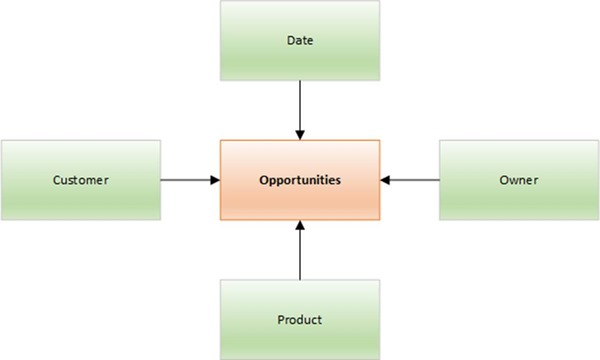When using Power BI, I typically create new data models from a data model template that includes a preconfigured Date table created using DAX. However, recently I needed to create a data model in Microsoft Excel for which I did not have a pre-configured data model template. It is possible to add an auto-generated Calendar table to the data model in Microsoft Excel. However, this table does not contain any columns for Fiscal Periods as shown here.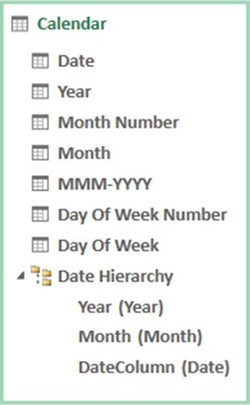I, therefore, decided to use Power Query to create a Date table containing the columns shown below. Some common columns in a Date table include Date, DateKey, Calendar Year, Calendar Quarter, Calendar Month, Fiscal Year, Fiscal Quarter and Fiscal Month.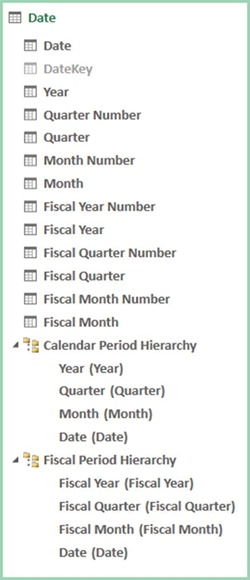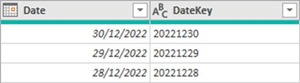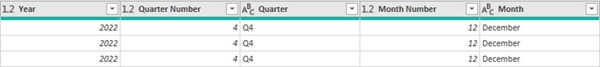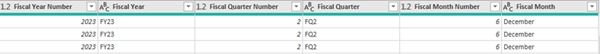The DateKey column is used for the configuration of relationships from the Date table to other tables in the data model. You can also configure such relationships using a Date column instead of a DateKey column. I use a DateKey column because this makes clear what the purpose of the column is and ensures that the format of the key values used by either side of the relationship is consistent.

There are separate Month and Fiscal Month columns because these will be pre-configured to be sorted by the Month Number and Fiscal Month Number columns respectively.

Parameters

The configuration of the query for this Date table includes the use of three parameters. They can be added using Manage Parameters in the Power Query editor window.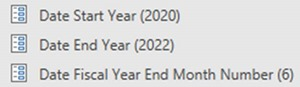Date Start Year and Date End Year are used by the Date table query to configure the range of dates in the Date table. You could replace these with Date Start Date and Date End Date parameters, however, you must ensure that the Date table always contains a full set of dates for all included years because the DAX Date/Time Intelligence functions you might use in any measures you add to the data model require this.

Date Fiscal Year End Month Number are used by the Date table query to configure the values for Fiscal Year Number, Fiscal Year, Fiscal Quarter Number, Fiscal Quarter and Fiscal Month Number. In this example, the Fiscal Year End is 30th June and so the Fiscal Year End Month Number is 6.

Applied Steps

These are the Applied Steps for the Date query.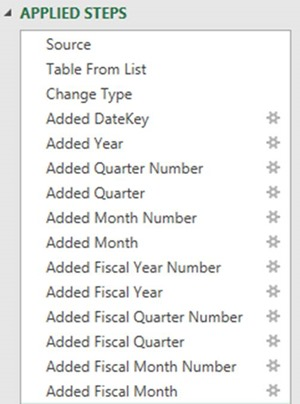Date and DateKey Columns

The Source step uses the List.Dates() function to generate a single column list of dates that are one day apart from each other starting from Date Start Date and ending after the number of days difference between Date End Date and Date Start Date. This step uses the Date Start Date and Date End Date parameters. In this example, the list contains 1,095 dates from 1/1/2020 to 31/12/2022. The list contains a single column named List.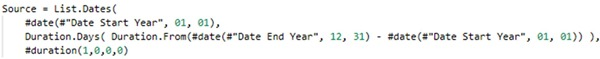Before any additional transformations can be applied to the list of dates, it must be converted to a table. The Table From List step uses the Table.FromList() and Splitter.SplitByNothing() functions to do this. The table contains a single column named Date.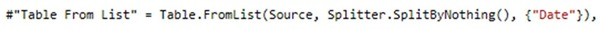The Change Type and Added DateKey steps use the Table.TransformColumnTypes() function to change the data type of the Date column from Any to Date and the Table.AddColumn() function to add a DateKey column. The DateKey for a Date such as 30/06/2022 is 20220630.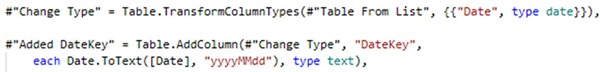Calendar Year Columns

The Added Year to Added Month steps use the Table.AddColumn() function to add the Year, Quarter Number, Quarter, Month Number and Month columns. Other functions such as Date.Year(), Date.QuarterOfYear(), Number.ToText(), Date.Month() and Date.ToText() are also used.

If required, the hard-coded prefix values as “Q” can be parameterized.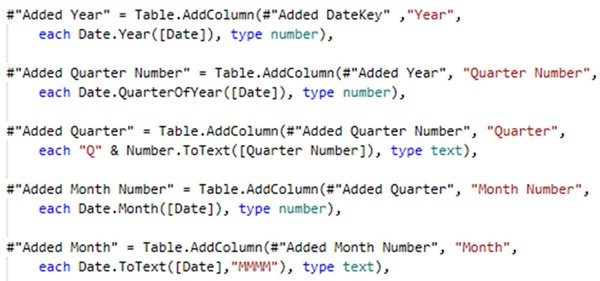Fiscal Year Columns

The Added Fiscal Year Number to Added Fiscal Quarter steps use the Table.AddColumn() function to add the Fiscal Year, Fiscal Quarter Number, Fiscal Quarter, Fiscal Month Number and Fiscal Month columns. Other functions such as Date.Year(), Date.QuarterOfYear(), Number.ToText(), Date.Month() and Text.End() are also used. Some of these steps use the Date Fiscal Year End Month Number parameter.

The Added Fiscal Quarter Number step is configured for a Fiscal Year End Month Number of either 3 (i.e., March) or 6 (i.e., June). If required, it can be modified to handle other Fiscal Year End Month Numbers and the hard-coded prefix values as “FY” and “FQ” can be parameterized.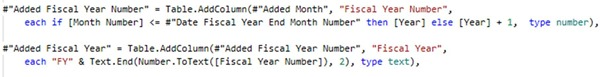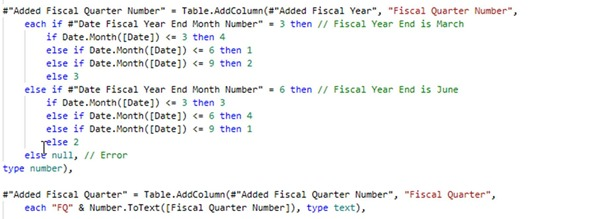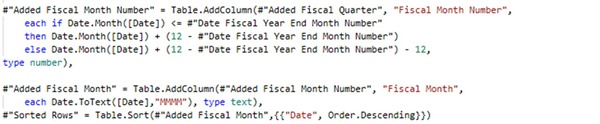Final Steps

Final steps for the configuration of this table are as follows:

Firstly, and most importantly, in the data model, select the Date table and mark it as a Date table using Mark as Date Table. The use of DAX Date/Time Intelligence functions in any measures you add to the data model require this.Secondly, and importantly, configure the Sort By Column for the Month and Fiscal Month columns to ensure that these are sorted by the Month Number and Fiscal Month Number columns.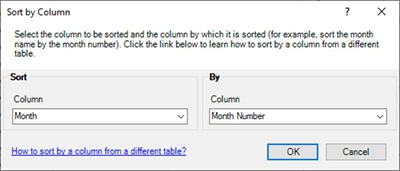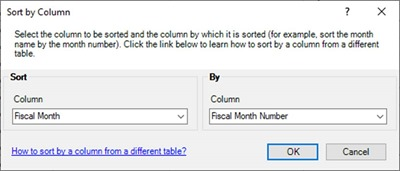Thirdly, and importantly, configure the relationships from the Date table to other tables in the data model. In this example, this requires the presence of corresponding DateKey columns in the other tables.

Fourthly, optionally configure a Calendar Period Hierarchy and/or Fiscal Period Hierarchy in the Date table.

Finally, ensure any Key columns such as DateKey are hidden in the data model.